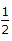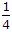# Civil Engineering - UPSC Civil Service Exam Questions - Discussion

5.

Two simply supported beams B1 and B2 have spans l and 2l respectively. Beam B1 has a cross-section of 1 x 1 units and beam B2 has a cross-section of 2 x 2 units. These beams are subjected to concentrated loads W each at the centre of their spans. The ratio of the maximum flexural stress in these beams is

 [A]. 4 [B]. 2 [C].[D].Explanation:

No answer description available for this question.

 Amlan Ghosh said: (Mar 18, 2014) fB1/fB2 = (MB1/i*y)/(MB2/i*y) = (wL/4ZB1)/(wL/2zB2) = Zb2/2Zb1 = 8/2 = 4.

 Rahul said: (Apr 3, 2014) M1 = Wl2/8, M2 = 4*Wl2/8 = Wl2/2, Z1 = 1/6, Z2 = 8/6, f1/f2 = (6*Wl2/8)/(6*Wl2/16) = 2.

 J.P said: (Jul 7, 2014) Stress = load/cross section area. W/A1=W/A2. W/1=W/4. 1=1/4. 4:1. Ans ..(a) 4.

 Saraba said: (Dec 8, 2015) M1 = wl/4; M2 = w(2l)/4 = wl/2; Z1 = (1*1^2)/6 = 1/6; Z2 = (2*2^2)/6 = 8/6 = 4/3; f1 = M1/Z1 = (wl/4)/(1/6) = 3wl/2; f2 = M2/Z2 = (wl/2)/(4/3) = 3wl/8; f1/f2 = (3wl/2)/(3wl/8) = 4 = 4:1.....(Answer)

 Shivam Chaudhary said: (Jan 11, 2016) To effectively compact chunks of clay the roller to be used is?

 Abhay said: (Jan 30, 2016) Stress = Load/Area. For B1 flexural stress = W/A1 = W/(1x1) = W. For B2 flexural stress = W/A2 = W/(2x2) = W/4. The ratio of the maximum flexural stress = W/(W/4) = 4.

 Rengel said: (Apr 24, 2016) Flexural stress should be equal to (3FL/2bd) right. Load/Area is the axial stress?

 Manesh said: (May 29, 2016) Stress= load/ c/s of area. There for B1 flex. Stress = load (w)/ (c/s of area) = w/1 = w. And B2 flex. Stress = load (w)/(c/s of area) = w/(2*2) = w/4. Then, the ratio of max flex stress= w/(w/4) = 4.

 Abiral said: (Jan 24, 2017) Stress = load/x - sectional area. For B1 flexural stress = w/1 * 1 = w/1, & for B2 flexural stress= w/2 * 2 = w/4, Hence, the ratio of max. flex stress = w/(w/4) = 4.

 Habtamu said: (Jul 25, 2017) It also be 1/4 you should specify the numerator and denominator to find the ratio. The question is some what ambiguous. If it is the of the flexural stress of smallest rectangle to the largest rectangle the answers is A. If vice-versa answers will be D.

 Avenso said: (Dec 30, 2021) Stress = load/x - sectional area. For B1 flexural stress = w/1 * 1 = w/1, & for B2 flexural stress= w/2 * 2 = w/4, Hence, the ratio of max. flex stress = w/(w/4) = 4.